# Complex Numbers in Polar Form

Complex numbers are written in polar (or trigonometric) form. The multiplications and divisions of complex numbers in polar forms are explained through examples and reinforced through questions with detailed solutions.

        

## Complex Numbers in Polar Form

Let us represent the complex number $$z = a + b i$$ where $$i = \sqrt{-1}$$ in the complex plane which is a system of rectangular axes, such that the real part $$a$$ is the coordinate on the horizontal axis and the imaginary part $$b$$ the coordinate on the vertical axis as shown below.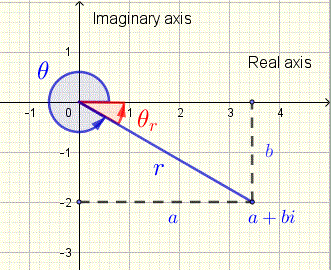where $$r = \sqrt {a^2 + b^2}$$ , $$r$$ is called the modulus of $$z$$
$$tan (\theta) = \left (\dfrac{b}{a} \right)$$ , such that $$0 \le \theta \lt 2\pi$$ , $$\theta$$ is called
argument of $$z$$.
The
reference angle $$\theta_r$$ to the argument $$\theta$$ is an acute angle made by the horizontal axis and the terminal side of $$\theta$$ and is defined as follows:
$$\theta_r = tan^{-1} \left|\dfrac{b}{a} \right|$$
If $$r$$ and $$\theta$$ are known, then $$a$$ and $$b$$ are given by
$$a = r \cos \theta$$
$$b = r \sin \theta$$
The complex number $$z = a + b i$$ may be expressed in polar form involving $$r$$ and $$\theta$$ as follows
$z = r (\cos(\theta)+ i \sin(\theta))$
Example 1
a) Plot the complex number $$z = 2\sqrt 3 - 2 i$$ on the complex plane and write it in polar form.
Solution to Example 1
See graph below of plot of $$z$$ on complex plane.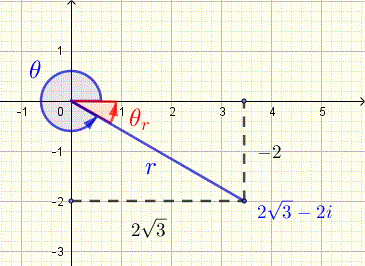$$r = \sqrt {(2\sqrt 3)^2 + (2)^2} = 4$$
We need to find the reference angle $$\theta_r$$ in order to find angle $$\theta$$.
$$\theta_r = \tan^{-1}\left|\dfrac{b}{a}\right| = \tan^{-1} \dfrac{\sqrt 3}{3} = \dfrac{\pi}{6}$$
The real part of $$z$$ is positive and its imaginary part is negative, hence the terminal side of the argument $$\theta$$ is in quadrant IV (see plot of $$z$$ above).
We now calculate $$\theta$$ as follows:
$$\theta = 2\pi - \theta_r = 2\pi - \dfrac{\pi}{6} = \dfrac{11\pi}{6}$$ (see plot of $$z$$)
$$z$$ in polar form is given by
$$z = 4 \left(\cos(\dfrac{11\pi}{6})+ i \sin(\dfrac{11\pi}{6}) \right)$$

Example 2
Write complex number $$z = \sqrt2(\cos(\dfrac{3\pi}{4})+ i \sin(\dfrac{3\pi}{4}))$$ in standard form.
Solution to Example 2
$$z = \sqrt2(\cos(\dfrac{3\pi}{4})+ i \sin(\dfrac{3\pi}{4}))$$
$$= \sqrt2(-\dfrac{\sqrt2}{2} + i \dfrac{\sqrt2}{2})$$
$$= - 1 + i$$

## Multiplication and Division of Complex Numbers in Polar Forms

Let $$z_1 = r_1 (\cos\theta_1 + i \sin\theta_1)$$ and $$z_2 = r_2 (\cos\theta_2 + i \sin\theta_2)$$ be complex numbers in polar form. Using the sum and difference trigonometric formula for sine and cosine, it can be shown that the product and quotient of these two complex numbers is given by
$$z_1 z_2 = r_1 r_2 (\cos(\theta_1+\theta_2) + i\sin(\theta_1+\theta_2))$$
$$\dfrac{z_1}{z_2} = \dfrac{r_1}{r_2} (\cos(\theta_1-\theta_2) + i\sin(\theta_1-\theta_2))$$

Example 3
Given $$z_1 = 3 (\cos(5\pi/4) + i \sin(5\pi/4))$$ and $$z_2 = (\cos(\pi/4) + i \sin(\pi/4))$$
Find $$z_1 z_2$$ and $$\dfrac{z_1}{z_2}$$
Solution to Example 3
$$z_1 z_2 = 3 (\cos(5\pi/4) + i \sin(5\pi/4)) (\cos(\pi/4) + i \sin(\pi/4)) = 3 (\cos(5\pi/4+\pi/4) + i \sin(5\pi/4+\pi/4))$$
$$= 3 (\cos(3\pi/2) + i \sin(3\pi/2))$$
$$= - 3 i$$
$$\dfrac{z_1}{z_2} = \dfrac{3 (\cos(5\pi/4) + i \sin(5\pi/4))} {(\cos(\pi/4) + i \sin(\pi/4))}$$
$$= 3 (\cos(5\pi/4-\pi/4) + i \sin(5\pi/4-\pi/4))$$
$$= 3 (\cos(pi) + i \sin(pi))$$
$$= - 3$$

## Questions

1) Write the following complex numbers in polar forms.
1. $$z_1 = i$$
2. $$z_2 = 2$$
3. $$z_3 = - 2 i$$
4. $$z_4 = - 3$$
5. $$z_5 = \sqrt3 + i$$
6. $$z_6 = - 3 - 3\sqrt3 i$$
7. $$z_7 = - 5 + 5 i$$
.

2) Use the results in part 1 above to evaluate the following expressions in polar form.
1. $$z_5 z_6$$
2. $$\dfrac{z_6 z_7}{z_5}$$
.

## Solutions to the Above Questions

1) Below are the plots of the complex numbers $$z_1, z_2, z_3, z_4$$ in questions a), b), c) and d). These plots help in explaining how is the argument found for each of these complex numbers that are either real (positive or negative) or pure imaginary (positive or negative imaginary part).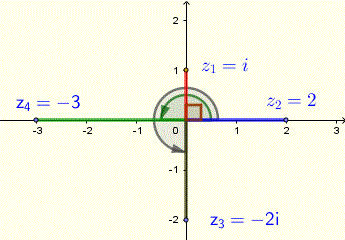1. $$z_1 = i$$
$$r = \sqrt{0^2+1^2} = 1$$
The plot of $$z_1 = i$$ on the complex plane gives a point at $$(0,1 )$$ with the terminal side of the corresponding argument (angle) on the vertical positive axis and hence $$\theta = \pi/2$$. (see plot above for argument and modulus)
$$z_1$$ in polar form is: $$z_1 = \cos ( \pi/2) + i \sin(\pi/2)$$

2. $$z_2 = 2$$
$$r = \sqrt{2^2+0^2} = 2$$
The plot of $$z_2 = 2$$ on the complex plane gives a point $$(2 , 0)$$ with the terminal side of the corresponding argument (angle) on the horizontal positive axis and hence $$\theta = 0$$. (see plot above for argument and modulus)
$$z_2$$ in polar form is: $$z_2 = 2 (\cos (0) + i \sin(0))$$
3. $$z_3 = - 2 i$$
$$z_3 = 2( \cos(3\pi/2) + i \ sin(3\pi/2))$$ (see plot above for argument and modulus)
4. $$z_4 = - 3$$
$$z_4 = 3 ( \cos(\pi) + i \ sin(\pi))$$ (see plot above for argument and modulus)
5. $$z_5 = \sqrt3 + i$$
$$r = \sqrt{(\sqrt3)^2+1^2} = 2$$
Find the reference angle $$\theta_r$$ defined as the angle between the horizontal axis and the terminal side of the argument $$\theta$$.
$$\theta_r = \tan^{-1}\left|\dfrac{b}{a}\right| = \tan^{-1} \left| 1 / \sqrt3 \right| = \pi/6$$
The real part and the imaginary parts are positive and therefore the terminal side of the argument is in quadrant I. Hence
$$\theta = \theta_r = \pi / 6$$
$$z_5 = 2 ( \cos(\pi/6) + i \ sin(\pi/6))$$
Plot of $$z_5$$ with more explanations is shown below.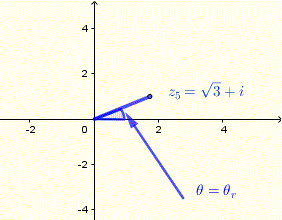6. $$z_6 = - 3 - 3\sqrt3 i$$
$$r = \sqrt{(-3)^2+(-3\sqrt3)^2} = 6$$
The reference angle $$\theta_r$$ defined as the angle between the horizontal axis and the terminal side of the argument $$\theta$$.
$$\theta_r = \tan^{-1}\left|\dfrac{b}{a}\right| = \tan^{-1} \left |(- 3\sqrt3) / (- 3) \right| = \pi/3$$
The real part and the imaginary parts are both negative and therefore the terminal side of the argument is in quadrant III. Hence
$$\theta = \pi + \theta_r = \pi + \pi/3 = 4 \pi/3$$
$$z_6 = 6 ( \cos(4\pi/3) + i \ sin(4 \pi/3))$$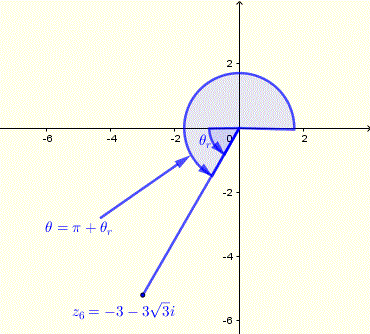7. $$z_7 = - 5 + 5 i$$
$$r = \sqrt{(-5)^2+(5)^2} = 5\sqrt2$$
Find the reference angle $$\theta_r$$
$$\theta_r = \tan^{-1}\left|\dfrac{b}{a}\right| = \tan^{-1} | 5 / - 5| = \tan^{-1} (1) = \pi/4$$

The real part is negative and the imaginary part is positive and therefore the terminal side of the argument is in quadrant II. Hence
$$\theta = \pi - \theta_r = \pi - \pi/4 = 3 \pi/4$$
$$z_7 = 5\sqrt2 ( \cos(3 \pi/4) + i \ sin(3 \pi/4))$$.

2)
1. $$z_5$$ and $$z_6$$ have already been expressed in polar form, we therefore use the formula for the product of two complex numbers in polar for given above.
$$z_5 z_6 = 2 ( \cos(\pi/6) + i \ sin(\pi/6)) \;\; 6 ( \cos(4\pi/3) + i \ sin(4 \pi/3))$$
$$= (2\times6)( \cos(\pi/6 + 4\pi/3 ) + i \ sin(\pi/6+4\pi/3)) = 12 ( \cos(3\pi/2) + i \ sin(\cos(3\pi/2) )$$
$$= - 12 i$$

2. Substitute $$z_5$$ , $$z_6$$ and $$z_7$$ by their polar form found above and use both formulas for the product in the numerator and the division
$$\dfrac{z_6 z_7}{z_5} = \dfrac{6 ( \cos(4\pi/3) + i \ sin(4 \pi/3)) \;\; 5\sqrt2 ( \cos(3 \pi/4) + i \ sin(3 \pi/4))}{2 ( \cos(\pi/6) + i \ sin(\pi/6))}$$

$$= \dfrac{ 30 \sqrt2 ( \cos(4\pi/3 + 3 \pi/4 ) + i \ sin(4 \pi/3+ 3 \pi/4 ))}{2 ( \cos(\pi/6) + i \ sin(\pi/6))}$$

$$= \dfrac{30 \sqrt2}{2} \sqrt2 ( \cos(4\pi/3 + 3 \pi/4 - \pi/6) + i \ sin(4 \pi/3+ 3 \pi/4 - \pi/6))$$

$$= 15 \sqrt2 ( \cos(23\pi/12) + i \ sin(23\pi/12))$$
.